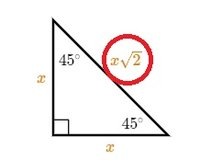# Square root of x squared?

#### Clydey

##### New member
I've decided to do something of a math refresher course, and it seems to be going well. However, I ran into a notation I didn't recognise, and I couldn't find it used elsewhere (I tried copy and pasting it into google). It seems to mean the square root of x squared, as far as I can tell. Can anyone confirm or supply an explanation? Here's the relevant image:Until I saw that image, I would have assumed that notation meant 2^x. Can anyone explain what I'm missing? It would be greatly appreciated.

#### MarkFL

##### Super Moderator
Staff member
Hello, and welcome to FMH!What that means is $$x$$ times $$\displaystyle \sqrt{2}$$. Personally, I would choose to write this as:

$$\displaystyle \sqrt{2}x$$

•topsquark

#### Clydey

##### New member
Hello, and welcome to FMH!What that means is $$x$$ times $$\displaystyle \sqrt{2}$$. Personally, I would choose to write this as:

$$\displaystyle \sqrt{2}x$$
Thanks for the response. That makes more sense. I should have thought of that. Presumably the index would be inside the v portion of the radical symbol?

#### MarkFL

##### Super Moderator
Staff member
Converting from radical to exponential form, we use:

$$\displaystyle \sqrt[n]{a^m}=a^{\frac{m}{n}}$$ where $$n\in\mathbb{N}$$ (this means $$n$$ is a natural number, or positive integer.)

•topsquark

#### Clydey

##### New member
Converting from radical to exponential form, we use:

$$\displaystyle \sqrt[n]{a^m}=a^{\frac{m}{n}}$$ where $$n\in\mathbb{N}$$ (this means $$n$$ is a natural number, or positive integer.)
Thanks for the insight. Much appreciated.

•MarkFL

#### Otis

##### Senior Member
... Presumably the index would be inside the v portion of the radical symbol?
Yes, that's where the index is shown.

When a radical expression displays no index, then the index is 2. In other words, a radical symbol with no index shown means square-root.

A side note: Be careful when handwriting radicals followed by a factor, to be sure it's clear what the radicand is (i.e., the expression inside the radical sign).

Even when properly formatted in printed material, I continue to see students read $$\sqrt{2}x$$ as $$\sqrt{2x}$$. With such expressions, I've gotten into the habit of always including explicit multiplication notation.

$$\sqrt{2} \cdot x\;$$ or $$\; \sqrt{2}\;(x) \;$$ or some such.

In fact, avoiding confusion could be the very reason why that author wrote $$x\sqrt{2}$$. Cheers•topsquark and MarkFL

#### pka

##### Elite Member
\sqrt[m]{x^n} wrapped in [_tex][/tex](remove the _) gives $$\displaystyle \sqrt[m]{x^n}$$

•MarkFL

#### MarkFL

##### Super Moderator
Staff member
\sqrt[m]{x^n} wrapped in [_tex][/tex](remove the _) gives $$\displaystyle \sqrt[m]{x^n}$$
You can wrap whatever you don't want to be parsed as BBCodes in PLAIN tags, for example:

[plain][MATH]\sqrt[m]{x^n}[/MATH][/plain]

This way, you can show users exactly what to post, as it renders thusly:

[MATH]\sqrt[m]{x^n}[/MATH]

•topsquark

#### topsquark

##### Full Member
Hmmm.. the noparse tag doesn't work here.

-Dan

#### mmm4444bot

##### Super Moderator
Staff member
You can wrap whatever you don't want to be parsed as BBCodes in PLAIN tags ...
Good example, Mark. For other members, the new no-parse feature and other helpful information can be found in this thread. Cheers•topsquark

#### Otis

##### Senior Member
Hmmm... the noparse tag doesn't work here.
Are you using [plain] and [/plain]?•topsquark

#### MarkFL

##### Super Moderator
Staff member
Are you using [plain] and [/plain]?Yes, that's XenForo's equivelent to vBulletin's noparse BBCode.

•topsquark

#### topsquark

##### Full Member
Are you using [plain] and [/plain]?I thanked that post but for some reason forgot about it. I was using noparse instead of plain.

-Dan

•MarkFL

#### Otis

##### Senior Member
... for some reason forgot about it ...
Maybe you suffer from CRS, like I do.#### topsquark

##### Full Member
Maybe you suffer from CRS, like I do.A "Career Retention Specialist?" Actually, as it happens my high school gym teacher was a real schmuck. I found out years later that he was in the Marines and was a DSI... "Drill Sergeant Instructor." He's the guy that makes the drill sergeants so nasty. I now figure we got off easy in gym class.

Seriously though. I found a number of possibilities for CRS and none of them looked nice. Makes my PTSD seem like a toy.

-Dan